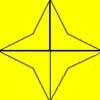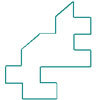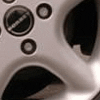#### You may also like### Star Find

Can you see which tile is the odd one out in this design? Using the basic tile, can you make a repeating pattern to decorate our wall?### Same Shapes

How can these shapes be cut in half to make two shapes the same shape and size? Can you find more than one way to do it?### Watch Those Wheels

Have you ever noticed the patterns in car wheel trims? These questions will make you look at car wheels in a different way!

# Posting Triangles

##### Age 5 to 7 Challenge Level:

Thank you for all your correct solutions. Three were particularly well explained.

Zeenat from St Margaret's Preparatory School wrote:

If the triangle is on the blue side then I found three ways to post it into the black hole. I used the dot to help me. I moved the dot to the three different corners of the triangle by turning the triangle and that is how I found three ways of posting the triangle into the black hole.
If I had to post it on both sides (blue and yellow) then there would be $6$ ways of doing it because I know that $3$ ways plus $3$ ways is equal to $6$ ways of doing it.

Mia from St Teresa's said:

I found six ways, three blue and three  yellow. The dot was at the top, then on the left and then on the right.

Amy from St Alban's Roman Catholic Primary School told us:

There are six ways.
The first you can just put the triangle in - $1$ way.
Then flip over the triangle to yellow - $2$ ways.
Then you turn the triangle so that the point is at the other corner - $3$ ways.
Turn the triangle as in number $3$ and flip the triangle yellow - $4$ ways.
Keep turning until the point is at the last corner - $5$ ways.
For the last time, turn it as in number $5$ and flip it yellow - $6$ ways.
That is all of the ways.